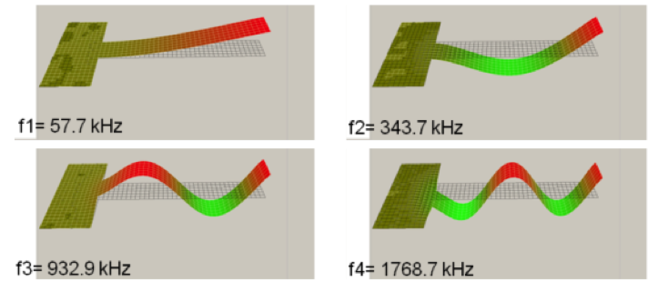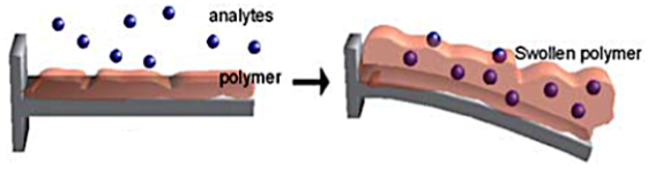## Want to keep learning?

This content is taken from the University of Twente's online course, Nanotechnology for Health: Innovative Designs for Medical Diagnosis. Join the course to learn more.
2.10

## University of Twente# How does it work? A physics model

In this step we explain the relation between the cantilever properties (dimensions, stiffness) and its vibrations. If you need further explanation of physical concepts such as frequency or oscillation I recommend to use Wikipedia. If you cannot follow the maths in detail, do not worry as it is not essential to understand the following steps.

A cantilever is a free-standing thin beam of material, that is clamped at only one end. In the electronic nose application the length of this beam is typically 20 to 200 $$\mu m$$, the width 10 to 50 $$\mu m$$, while the thickness is only a few $$\mu m$$.

A cantilever works much the same as the diving board, that you probably know from your local swimming pool. You know that the board moves up and down with a certain vibration frequency when you jump up and down at the end of the board.

This specific frequency is called an eigen frequency (eigen is German for self), because it is determined by the properties of the board itself. For frequencies close or equal to the eigen frequency the amplitude of the oscillation becomes very large, much larger than for other frequencies. When vibrating at this eigen frequency, the cantilever is said to be in resonance.Measured 1st to 4th eigen modes $$ψ_n$$ of a cantilever with eigen frequencies $$f_1$$ to $$f_4$$ (Cantilevers fabricated and measured by Dr. Minh Nguyen, University of Twente.) © University of Twente

The cantilever can vibrate at different eigen frequencies in different eigen modes. In a simple model the eigen frequencies $$f_n$$ are given by the formula:

$f_n=\frac{α_n t}{L^2}\sqrt{\frac{E}{\rho}}$

$$t$$ and $$L$$ are the thickness and length of the cantilever; $$E$$ is the Young’s modulus or elasticity modulus (a measure of the stiffness of the cantilever: the larger this number the stiffer the beam) and $$\rho$$ is the mass density (kg/m3) of the beam material. The number $$α_n$$ depends on the mode number $$n$$ ($$α_1$$=6.41, $$α_2$$=39.90, $$α_3$$=111.8, $$α_4$$=219.5).

The shapes of the eigen modes are described by mathematical functions $$ψ_n (x⁄L)$$. The cantilever is clamped at the end with $$x$$=0. The shape (deflection) of the oscillating cantilever for a given eigen frequency is the function $$z(x)=Aψ_n (x⁄L)$$, that gives the deflection of the cantilever in the $$z$$-direction at position $$x$$ along the cantilever. The oscillation amplitude $$A$$ depends on how hard it is driven (in terms of the diving board, how hard the swimmer jumps up and down to push the board deeper down). With increasing number $$n$$ more and more deformation (bending) of the cantilever occurs.

### Changing cantilever properties - effect on $$f_n$$

From the first equation it is clear that $$f_n$$ can be changed by changing the beam dimensions (length $$L$$ and thickness $$t$$) and the beam material properties (mass density $$\rho$$ or Young’s modulus $$E$$). Once a cantilever is designed and fabricated the dimensions are of course fixed. However one may change in some way the $$\rho$$ or $$E$$. We can rearrange the formula to bring out the dependence on the cantilever mass $$m=ρtWL$$ more clearly, ($$W$$ is the width of the cantilever)

$f_n=α_n \sqrt{\frac{t^3 W}{L^3 }} \sqrt{\frac{E}{m}}$

The second equation tells us that if the cantilever mass is increased by $$Δm$$ (keeping the other parameters the same), then the eigen frequency decreases. Thus if we are able in some way to have a specific amount of VOC material attach to the cantilever, then we can calculate from the frequency shift the added mass and thus the amount of VOC, that is attached.

On the other hand as the VOC molecules attach, forming an additional thin layer, this itself has a certain stiffness, that changes the overall stiffness of the cantilever by a certain amount $$ΔE$$. In practice we observe that the cantilever always becomes stiffer, when a layer is added, thus the resonance frequency increases due to adsorption of the VOC. Another way to employ the effect of the change in stiffness is to use an organic layer that is sensitive for the absorption of a specific VOC. In the process of absorption the VOC is incorporated in the layer. After absorption the elastic properties of the organic layer have changed (increased or decreased) and thus the resonance frequency of the cantilever changes.The value of the eigen frequency is very sensitive to mass and stiffness changes: the cantilever has become a mass and/or stiffness sensor.

When the deposited layer adds to both the mass and the stiffness then the frequency change is due to the combined effects. The net frequency change may even be zero!

A possible approach to tackle this problem is to have the added material attach only on specific positions on the cantilever. It can be shown that the cantilever eigen frequency is most sensitive to mass changes if the added mass is positioned on the cantilever where the amplitude $$z(x)=Aψ_n (x⁄L)$$ is largest. The frequency change is negligible where the amplitude is small. In contrast, the frequency change due to an additional elastic layer is largest when the material is deposited on the positions with the highest slope, (at the points where the amplitude is small) and the response is small where the amplitude is largest.

Thus the cantilever can be made more $$∆m$$-sensitive or more $$∆E$$-sensitive by making the cantilever VOC-sensitive at specific positions. In this way two parameters can be determined simultaneously, which may allow for a better differentiation between different VOCs.